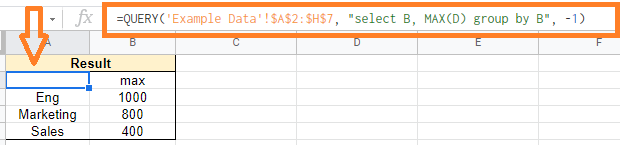Google Sheets QUERY Function Explained With Examples

Query Function in Google Sheets helps us to extract the specific set of data from a tabular column. For example, let’s say you have a huge employee dataset of your company and you would like to view only marketing team details. In this case, you can use the Query Function to extract the department’s dataset.

The query function can also be used to extract a subset of data from the overall dataset. For example, if your data includes eleven columns, you can utilize Google Sheets’ QUERY function to extract only four or five of them.

The query function syntax which needs to be used in Google Sheets are given below:

• data: The range of cells on which the query will be run. Only boolean, numeric (including date/time types), or text values are allowed in each column of data. In the event of multiple data types in a single column, the data type of the column for query purposes is determined by the majority data type. Null values are assigned to data types that are in the minority.
• query: This is the query expression you will use to get the data you need. The Google Visualization API Query Language was used to create this query expression. This is also quite similar to how SQL queries are expressed. This is always enclosed in double quotation marks.
• headers: This parameter is optional. The number of rows in the header at the top of the data. If this parameter is left blank or set to -1, the value is calculated based on the data.

Understanding Query Function in Google Sheets with Examples

In order to understand the query function in Google Sheets, let’s consider the following dataset.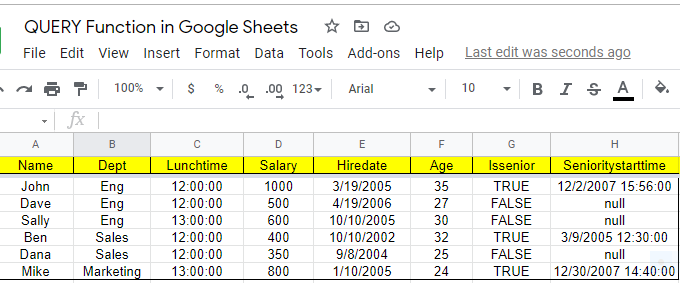In the above dataset, we have an employee dataset and now let’s use the Query function to perform various operations

Extracting Selected Columns in Google Sheets using Query Function

In our case, we are using the same dataset, which is visualized in the above section. Here we want to extract the employee name and their department.

Follow the steps listed below to extract the columns in Google Sheets using the Query function.

• Step 1: Open the Google Spreadsheet where you want to use the Query function.
• Step 2: Now, to extract the columns, we should use the SELECT expression.
• Step 3: The formula to extract the employee name and their department id is “=QUERY(Example Data, “Select A, B”). The Example Data is our sheet name.
• Step 4: Press the enter button.

You will see the results.Extract Data in Ascending or Descending Order using QUERY Function in Google Sheets

While extracting the data using the QUERY function, we can also specify the order such as ascending or descending.

For example, in the above example, let’s see how the query function extracts the data from the employee department and their salary.

• Step 1: Move to the cell where you would like to extract the data in ascending or descending order using the QUERY function.
• Step 2: Now to extract the data in ascending order, use the formula as =QUERY(Example Data, “Select B, D Order by D Asc”).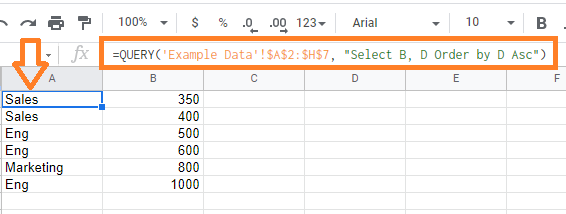• Step 3: To extract the data using a descending order, use the formula as =QUERY(Example Data, “Select B, D Order by D Desc”)
• Step 4: Press the “Enter” button and you will see results.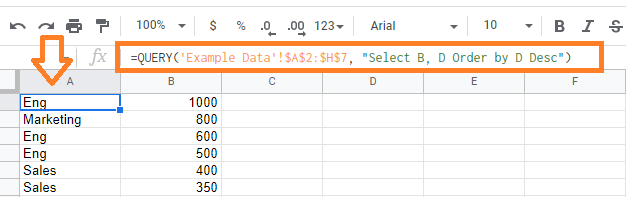Extract Data Which Meets Conditions using QUERY Function

Using the Select and Where clauses, the QUERY function returns rows that fit the provided condition.

In this example, I would like to extract the data where the employee is not falling under the engineering category and is senior or older than other employees in column A.

Let’s understand how to use the QUERY function to achieve this by following the steps listed below:

• Step 1: Move to the cell where you would like to extract the data.
• Step 2: Now enter the formula “=QUERY(‘Example Data’!\$A\$2:\$H\$7, “select A where (B<>’Eng’ and G=true) or (D > “&A2&”)”)“.
• Step 3: Press the “Enter” button.

You will see the results being extracted in the Google Sheets.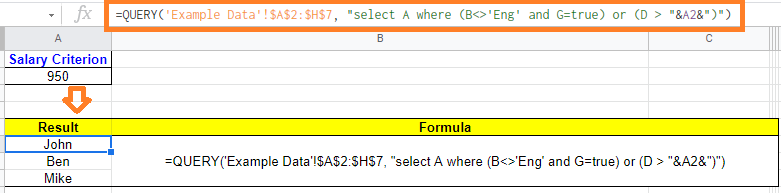Group the Data using QUERY Function in Google Sheets

Suppose, in this example, I want to extract the maximum salary of each department’s employee. To achieve this, we can use the QUERY function. The steps to achieve this are explained below:

• Step 1: Select the cell where you would extract the results.
• Step 2: Now enter the formula “=QUERY(‘Example Data’!\$A\$2:\$H\$7, “select B, MAX(D) group by B”)“.
• Step 3: Press the “Enter” button.